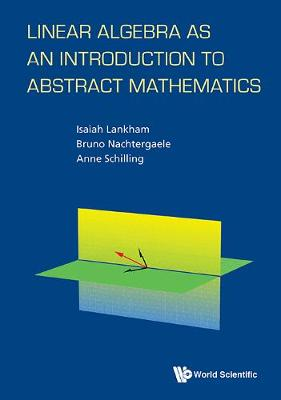•# Linear Algebra As An Introduction To Abstract Mathematics (Paperback)

(author), (author), (author)
£30.00
Paperback 208 Pages / Published: 28/01/2016
• We can order this

Usually dispatched within 1 week

This is an introductory textbook designed for undergraduate mathematics majors with an emphasis on abstraction and in particular, the concept of proofs in the setting of linear algebra. Typically such a student would have taken calculus, though the only prerequisite is suitable mathematical grounding. The purpose of this book is to bridge the gap between the more conceptual and computational oriented undergraduate classes to the more abstract oriented classes. The book begins with systems of linear equations and complex numbers, then relates these to the abstract notion of linear maps on finite-dimensional vector spaces, and covers diagonalization, eigenspaces, determinants, and the Spectral Theorem. Each chapter concludes with both proof-writing and computational exercises.

Publisher: World Scientific Publishing Co Pte Ltd
ISBN: 9789814723770
Number of pages: 208
Weight: 454 g
Dimensions: 241 x 170 x 15 mm